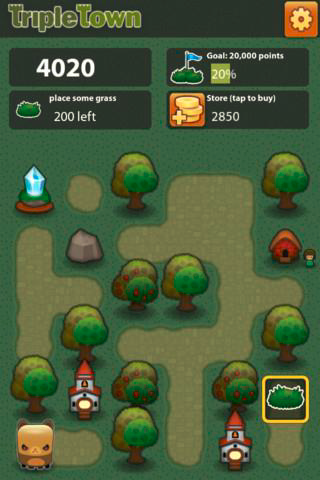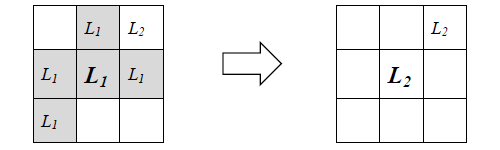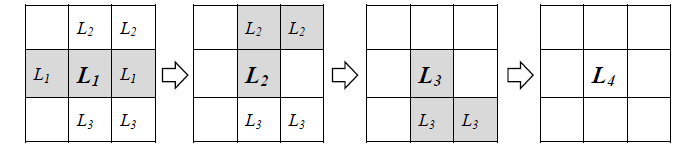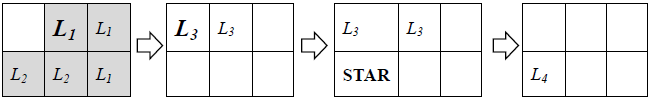## Day 2, Problem 3 - Triple Town

XiaoXi has recently been addicted to the popular iOS game Triple Town. Outside of just playing, XiaoXi has also been thinking about how to obtain higher scores in this game.

As depicted in figure 1, the game takes place on an n×m map. The game will provide a certain build sequence of tiles, which the player must follow and select empty squares to build these corresponding tile. Building tiles are divided up into nine different levels. In ascending order of level, they are grass, bush, tree, hut, …, etc. (to make our description easier, we will simply refer to them as L1, L2, L3, …, L9).Figure 1.

After a player has built a tile on an empty square, a reaction may take place. The conditions for a reaction to form is: starting from the current square, if the total number of connected squares which has the same level is greater than or equal to 3, then this entire connected region will merge into a single, more advanced tile that is one level higher. The other tiles connected to the current tile will turn back into empty squares. Here, connected tiles refer to the set of all tiles that are directly adjacent or indirectly connected by other adjacent tiles. Another issue to note is, L9 is the highest possible level for a tile. Multiple L9 tiles will not merged if connected. For example in figure 2, after building the center L1 tile, all the connected blocks will merge together to form a single L2 tile.Figure 2.

Note: in the process of merging tiles, chain reactions may occur. This is depicted below in figure 3.Figure 3.

The scoring of the game depends on the tiles and their levels, as built by the player or produced by reactions. The score distribution for the different leveled tiles are as follows:

 Tile Level Score Value L1 L2 L3 L4 L5 L6 L7 L8 L9 4 20 100 500 1,500 5,000 20,000 100,000 500,000

Take the two scenarios depicted above for example. In figure 2, an L1 tile was built to produce a score of 4. Afterwards, L1 tiles merged to produce an L2 tile, thus generating a score of 20. In total, the total score sums to 24. In figure 3's scenario, the single move would yield a score of 4 + 20 + 100 + 500 = 624.

To reduce the difficulty of the game, two different tools respectively called "stars" and "bombs" were introduced. At the start of the game, the player is given p stars and q bombs. The player can use these tools at any moment. Their functions are as follows:

Stars A star may be placed on any empty square. When a star is placed, it will automatically transform into a tile capable of invoking the highest possible level of reaction. However when no reactions may be formed at this location, the star will automatically transform into a L1 tile. For example, if a star were placed (instead of the L1) in the center of the grid, then it would automatically transform into an L3 tile. The scoring afterwards would behave normally as if an L3 tile was placed. A bomb may be placed on any square that's already occupied by a tile. When a bomb is placed, the tile currently at that square will be destroyed and it will be reverted back to an empty space. When using a bomb, half of the value of the destroyed tile will be deducted from the player's score (i.e. a negative score will be incurred).

In the process of the game, the player must build tiles in the given fixed sequence, and may use these tool at any time. The objective of the game is, through appropriate build operations, obtain the highest score possible.

To help students better understand this game, XiaoXi has also written a very simple demo program that is available to you for experimentation.

### Input Format

There are 10 test cases `tritown1.in` ~ `tritown10.in` that will be given to your program (through standard input). They can be downloaded here for you to study: tritown.zip

Line 1 of input contains an integer from 1 to 10, representing the test case number. Test case `tritowni.in` will have i on its first line.
Line 2 will contain two integers n and m, representing the number of rows and columns in the game map.
Line 3 will contain two integers p and q, respectively representing the total number of stars and bombs at your disposal.
The following n lines will contain an n×m grid, representing the initial status of the map. Here, the character '`.`' represents an empty space, while a number between 1 and 9 represents the level of an already placed tile.
The following line will contain a single integer k, representing the length of the build sequence.
The last line will contain k space-separated integers between 1 and 9, representing the levels of every tile in the build sequence, in the order that should be built.

### Output Format

Every line should consist of a player command in one of the 4 formats below:

CommandMeaning
`PUT x y` Place the next tile in the build sequence on the empty space at row x, column y.
`STAR x y` Place a star on the empty space at row x, column y.
`BOMBER x y` Place a bomb at row x, column y. This location must be occupied by a tile.
`END` Game over. Count the current score.

```0
2 3
1 1
..1
221
2
1 3
```

### Sample Output

```PUT 1 2
PUT 1 1
STAR 2 1
END
```

### ExplanationThe first move scores 4 + 20 + 100 = 124 points.
The second move scores 100 points.
The third move scores 100 + 500 = 600 points.
The total score of the game is 124 + 100 + 600 = 824.

### Scoring

For each test case, we have set 9 grading parameters a10, a9, …, a2. If your solution is invalid or does not fit the requirements, then you will be given a score of zero. Otherwise, let wuser represent the number of points you obtain with your solution strategy. Your score out of 10 for the test case will be determined follows:

ScoreCondition
10wusera10
9wusera9
8wusera8
7wusera7
6wusera6
5wusera5
4wusera4
3wusera3
2wusera2
1wuser > 0

If multiple conditions are satisfied, then the condition that yields the highest score will be taken.

### Experimentation

We supply a tool tritown_check.py for you to test whether your solutions are accepted. The usage for this program is:

`tritown_check.py <input-file> <output-file>`

When you execute `tritown_check.py`, the program will process your supplied input and output files and print the results to standard output. Possible results of the execution includes:

• Abnormal termination: An unknown error occurred.
• `Input/Output File Does Not Exist!`: We cannot load your input or output file.
• `Output Invalid!`: There is an error with your output file. A general error message may now be included.
• `Correct! Your score is x`: Your output is acceptable. The amount of points obtained in the game by your solution strategy is x.

Point Value: 40 (partial)
Time Limit: 10.00s
Memory Limit: 512M